top of page
Search

# FEA Brain Teasers - FEA Exam And Interview Prep - Analysis

Welcome to the final post in our three part series featuring all of the Brain Teasers that were posted weekly on the Fidelis LinkedIn page between 2020 and 2022!

This week’s collection of Brain Teasers focuses on analysis-related questions, further sorted by subject. The answers to these questions, as well as the explanations, are shown at the bottom of the post. These Brain Teasers make for excellent practice for technical interviews or for anyone looking to reinforce their FEA knowledge. Let us know how many you get right!

Part 1: Materials

Part 2: Mechanics

## Questions

### General Concepts

1.2.### Element Types

3.4.5.6.### Element Selection

7.8.9.### Element Quality

10.11.12.13.### Model Setup

14.15.16.17.### Model Checks

18.19.20.### Boundary Conditions

21.22.23.### Nonlinearity

24.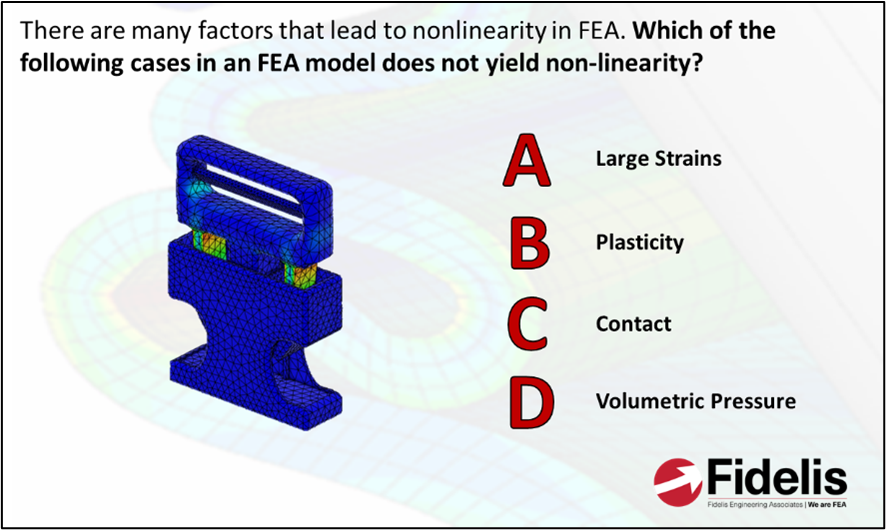25.### Contact

26.27.28.29.30.### Analysis Type

31.32.33.34.### Thermal Analysis

35.36.37.### Unit Continuity

38.39.40.1.In this equation, K is known as the stiffness matrix, u is the vector of displacements, and F is the vector of forces. Check out this blog to learn more! https://www.fidelisfea.com/post/what-is-finite-element-analysis-and-how-does-it-really-work

2.Discretization is the process of breaking up a problem with infinite degrees of freedom into one with finite degrees of freedom... hence the term 'finite element analysis. Although it is also typical to keep our analyses secret, especially when there are NDAs involved...

3.Being constant strain elements, first-order tetrahedrals tend to exhibit overly stiff behavior, particularly when they are used in relatively coarse mesh. In order to overcome this issue, it is necessary either to utilize very fine mesh or second-order elements.

4.This was a very tricky question! Obviously, when looking at one element alone, first order has 4 nodes and second order has 10 - so the ratio in a single element is 2.5 times. However, when you start to arrange elements into a mesh, the sharing of the corner and edge nodes is very different. You can see in the image above that corner nodes (blue) of a triangular mesh (easier to visualize) share 6 elements, while edge nodes (red) share only 2. So, although the ratio between first and second order is exactly 2 in a single element (3 to 6), we need more than double the number of nodes to convert our MESH to second order. In a 3D, tetrahedral mesh, this effect is also observed, resulting in a ~6 times increase in node count just for converting from first to second order mesh.

5.Trussed structures tend to take advantage of the axial stiffness of thin members, rendering the bending stiffness essentially negligible. Hence, in FEA, we typically ignore them to save on computational expense.

6.Plane frame members can be subjected to in-plane loads that can cause axial, shear, and bending stresses, hence the degrees of freedom at a node of a plane frame element are U, V, and θz.

7.We're assuming no existing damage and the vessel is thin-walled, so only membrane stresses are really relevant here, which leads us to our shell element conclusion. Using axisymmetric elements might be oversimplifying the loading a little bit (we can't include the cradle or gravity) as well as requiring a lot of elements to properly capture wall thickness while maintaining aspect ratio. If you wanted to use 3D elements, again, you'd need a lot of them and would likely end up with a model that is much too large to run efficiently (or even at all). Neither are impossible, just not the optimal approach in our experience.

8.Since incompressible materials do not change in volume when loaded in compression, the pressure stress associated with this loading type cannot be computed in traditional finite elements. Hybrid elements include an additional degree of freedom that determines the pressure stress directly. The nodal displacements are used only to calculate the deviatoric strains and stresses.

9.Plane stress is great for analyzing very thin plates that are only loaded in the plane, but it can also be applied to the surface of thicker components. Check out this blog to learn more! https://www.fidelisfea.com/post/plane-stress-vs-plane-strain-what-are-they-and-which-should-you-choose

10.Warpage measures the ‘out of planeness’ of a quad element by way of factor or angle. As the image above shows, this is only relevant in quad type elements since triangles can only ever exist in a single plane.

11.Shear locking can occur in first-order, fully-integrated elements (C3D8) that are subjected to bending. This happens when artificial shear strain develops due to an inability of the element edges to bend. As a result of this nonphysical shear strain, these elements can be too stiff when used in bending-dominant problems.

12.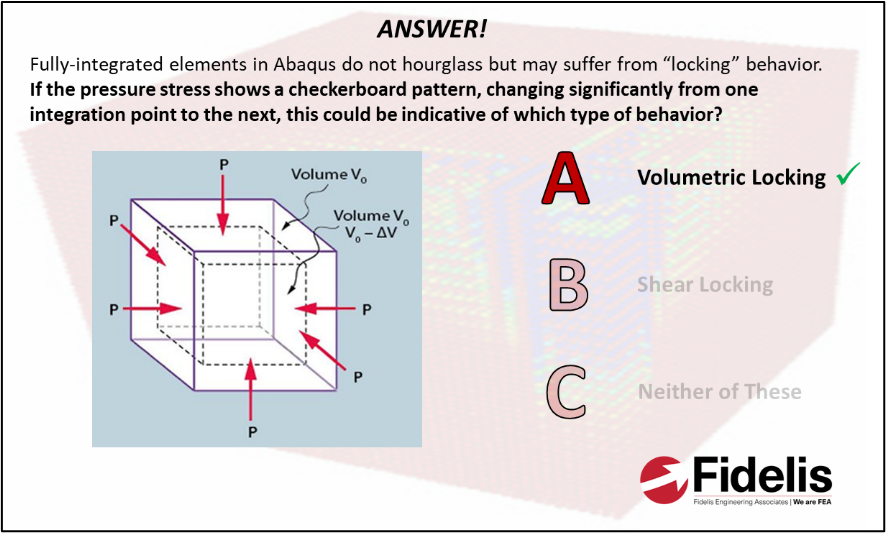In Abaqus, volumetric locking occurs in second-order, fully integrated elements when the material behavior is (nearly) incompressible. This results in overly stiff behavior for deformations that should cause no volume changes.

13.Being reduced integration elements, the coarse mesh results in excitation of zero-energy degrees of freedom and hourglassing is seen. In Layman's terms, the elements can change shape under bending without causing any measurable stress or strains. In Abaqus, the beam becomes less stiff and shows a deflection of 2.8mm, where the handbook solution suggests 2.0mm. That's a 40% error!

14.In a kinematic coupling (RBE2), the 'control node' drives the motion of the dependent nodes, which gives us rigid behavior, whereas in a distributing coupling (RBE3), the solver is averaging the behavior of many nodes in order to determine the response at a single node. This contributes no added stiffness to the model. For something as stiff as an engine, kinematic couplings would be more suitable.

15.In short, shell-to-solid couplings create distributing couplings to the solid for each node in the shell to account for the shell's thickness. Tie constraints simply tie the nodes on the shell to the closest nodes in the solid, leading to artificial stress concentrations. Meanwhile, kinematic couplings would provide overly stiff behavior in the middle of the part.

16.Plane strain assumptions tend to be a very good approximation of the behavior inside a thick component that is loaded only in one plane. The large amount of material through the thickness of the dam essentially renders through-thickness strain irrelevant (or at least negligible).

17.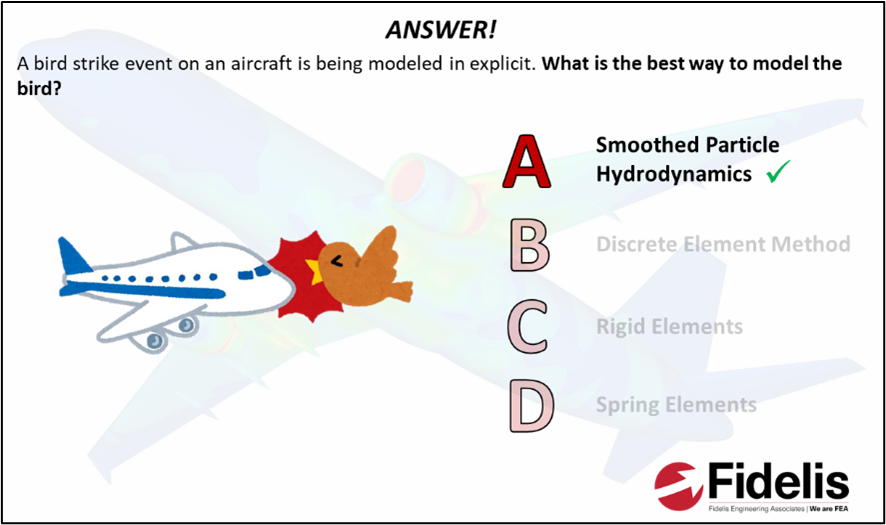That poor bird… since the bird has a very high deformability, SPH is a good model choice since it is a mesh-free method and has a low computational cost.

18.Stress cannot be resolved at a singularity – no matter how many mesh convergence iterations you run! Here we have a stress singularity at a perfectly sharp reentrant corner, which would result in a theoretical stress of tending toward infinity with reducing mesh size. Of course, this can never happen in reality, but it's always a challenge to decide just how small of a filet you're willing to include in an FEA model.

19.There is also an argument that stress profiles in some scenarios could end up being inaccurate, but not necessarily exactly inverse.

20.Although not the only cause of numerical singularities, rigid body motion is by far the most common. Numerical singularities occur where there is no mathematical resolution for the degree of freedom (DOF) of the particular node. In most cases, this is because static equilibrium cannot be resolved in a moving body - the analysis is under constrained.

21.The nodes of 3D elements only have active degrees of freedom translationally (1-3), hence the single node was not constrained from spinning. As we can see from the snippet of the message file, the job failed to run due to numerical singularities (rigid body motion).

22.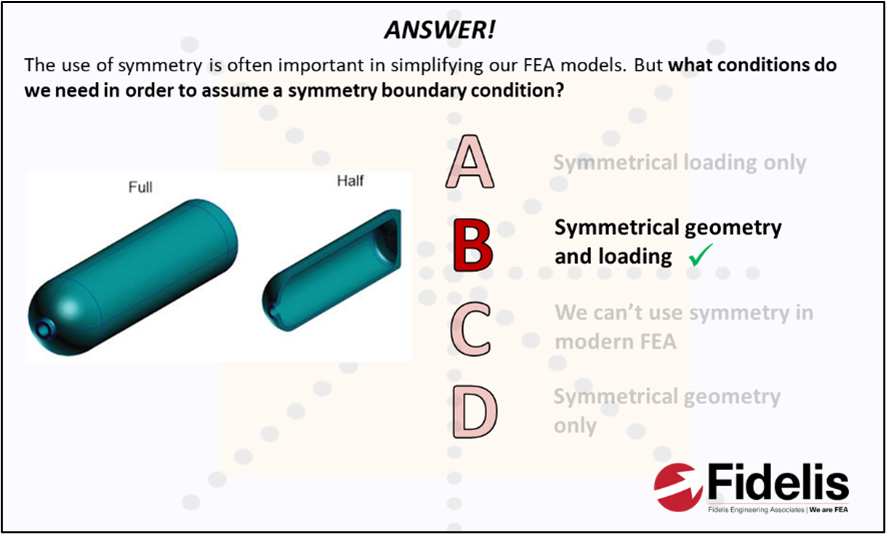There are also two more requirements: material behavior and expected mode of deformation. Typically, isotropic materials only (but that's not a hard and fast rule) and symmetric deformation (careful of buckling and mode shapes) must also be ensured.

23.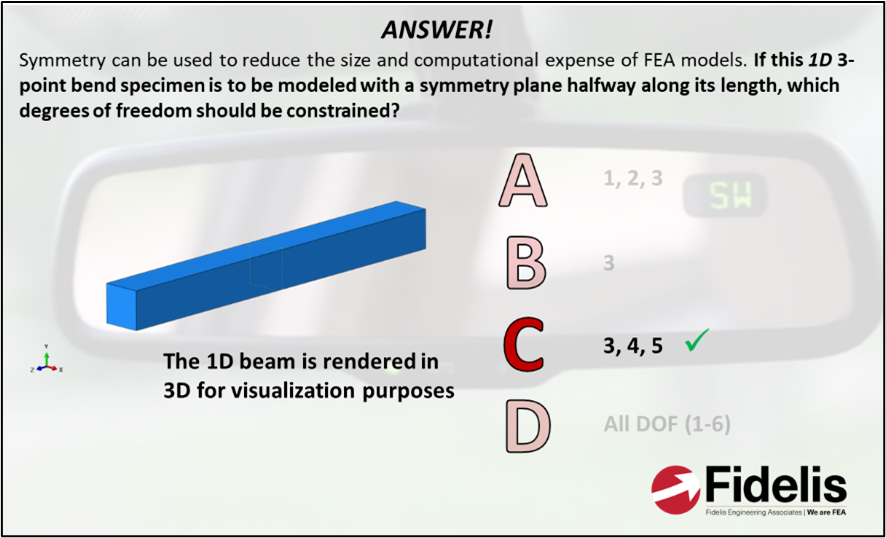In this particular model, we're looking to model symmetry in the XY plane, hence we constrain in the Z direction translationally and then about X and Y rotationally.

24.The three main sources of nonlinearity in FEA are geometric, material, and contact, which are represented by choices A, B, and C respectively.

25.In a nonlinear analysis the solution usually cannot be calculated by solving a single system of equations, as would be done in a linear problem. Instead, the solution is found by applying the specified loads gradually and incrementally working toward the final solution. The Newton-Raphson method is a root-finding algorithm which can efficiently approximate the roots of a function, making it ideal to solve nonlinear analyses.

26.In node to surface contact, the slave nodes are constrained not to penetrate into the master surface; however, the nodes of the master surface can penetrate into the slave. Surface to surface contact, however, enforces contact conditions in an average sense over the region nearby the slave nodes – rather than only at individual slave nodes. This results in more realistic pressure and stress results local to the contact.

27.The reason for the slave being finer than the master is that the master nodes are allowed to penetrate the slave surface, hence we try to keep the slave surface finer to reduce the effect on the contact solution.

28.The reason we shouldn't blindly trust results at any other time during the step is because artificial energy is introduced to help stabilize contact. This essentially resists rigid body motion and allows static equilibrium to be found; even where it doesn't/shouldn't exist. However, once stabilization energy is ramped to zero at the end of the step, results will be valid.

29.As a bolt is preloaded, it stretches and the parts being clamped compress. When external load is applied, the bolt will begin to stretch, but the compressive forces will also relax, effectively meaning the load is shared. Once the preload is completely removed (not good for your connection), the bolt takes all remaining external load that is applied.

30.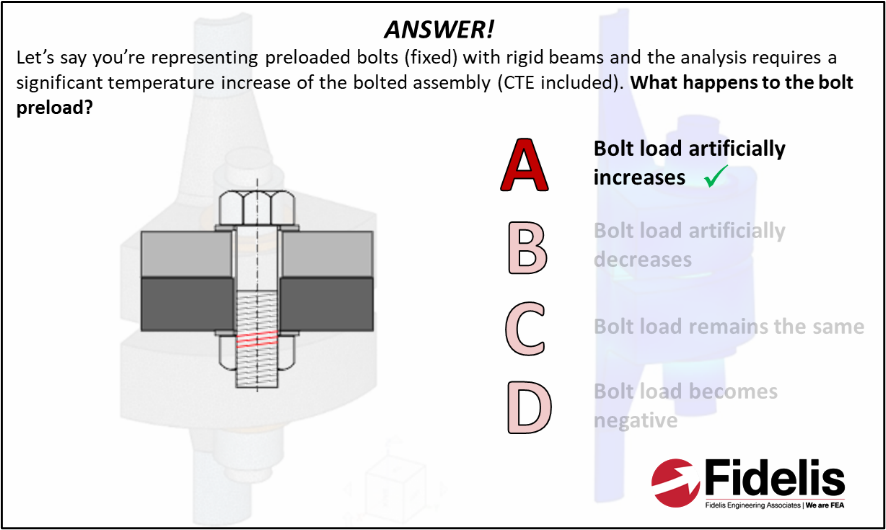If the bolted material expands and the bolt is rigid, then the bolt force will increase artificially. If the bolt was modeled with fully fidelity, it would also expand and the preload would be relevant to the case being simulated.

31.When strain rates are very high, explicit analysis should be used instead because it captures the inertial effects of high strain rate problems.

32.In this case, we are trying to mimic the static equilibrium that we find in a standard/implicit analysis by reducing the ratio between internal energy and kinetic energy. As long as kinetic energy is low enough (things aren't flying around too fast), we can make the assumption that the system is behaving in a static manner. To do this, we can either apply mass or time scaling (or both).

33.The maximum stable time increment is defined by the Courant time step (CFL number). That is the time taken by a sound wave to travel across an element and is defined by the element’s size, material stiffness, and material density.

34.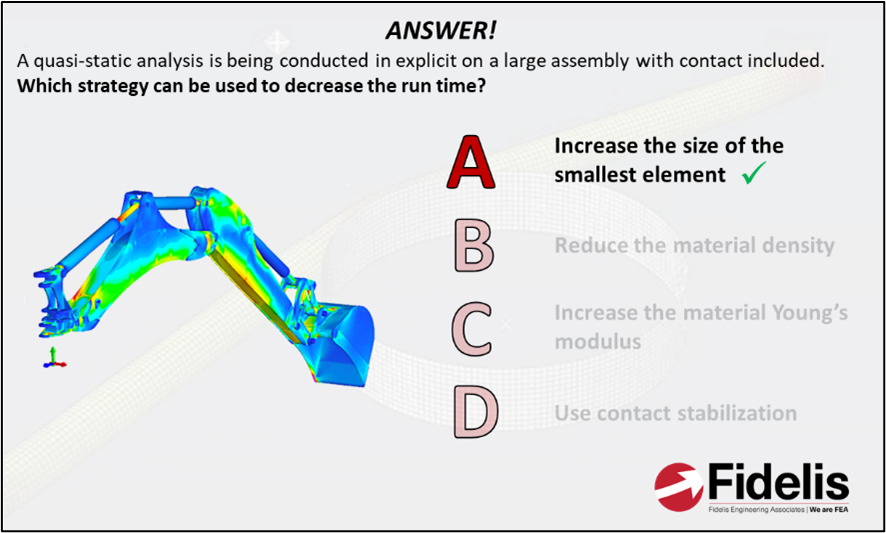Mathematically, the critical time step is the ratio of characteristic element length and wave speed. The wave speed is approximated as sqrt(E/rho). So, by increasing the size of the smallest element, the critical time step can be increased which will result in a decrease in the run time.

35.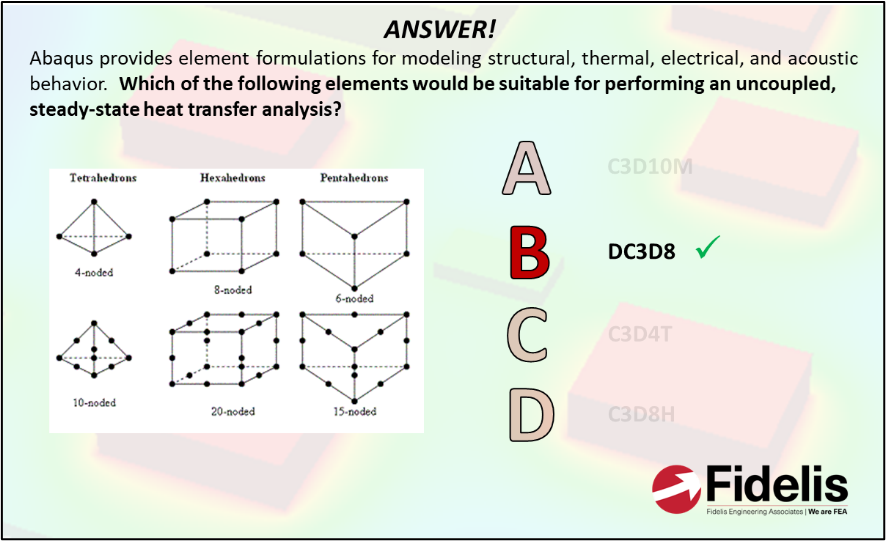DC3Dx elements for steady-state thermal analysis - C3DxT elements are actually for fully-coupled, thermomechanical analysis.

36.The Stefan-Boltzmann constant is a constant of proportionality that states the total intensity radiated over all wavelengths increases as the temperature increases.

37.Since the mechanical behavior of the system has almost no impact on the results of the thermal analysis, we can avoid fully coupled analysis, which is typically more expensive.

38.Length, mass, and time are the only units here that we can consider 'base'. They are not derived from any other units - they are simply measurements. Derived units, which the rest are, come from combinations of other units (e.g. stress is derived from force/area).

39.To keep the unit system consistent to inches and seconds, we need to define mass in blobs! If you want to learn more about consistent unit systems in FEA, check out or cleverly named blog post on the topic: https://www.fidelisfea.com/post/understanding-units-in-fea-what-in-the-blob-is-a-slug

40.Maybe a confusing question, but we're saying that frequencies decrease because mass is wildly over-stated when you accidentally assume density should be defined in g/mm3 rather than tonne/mm3.

1,691 views
bottom of page